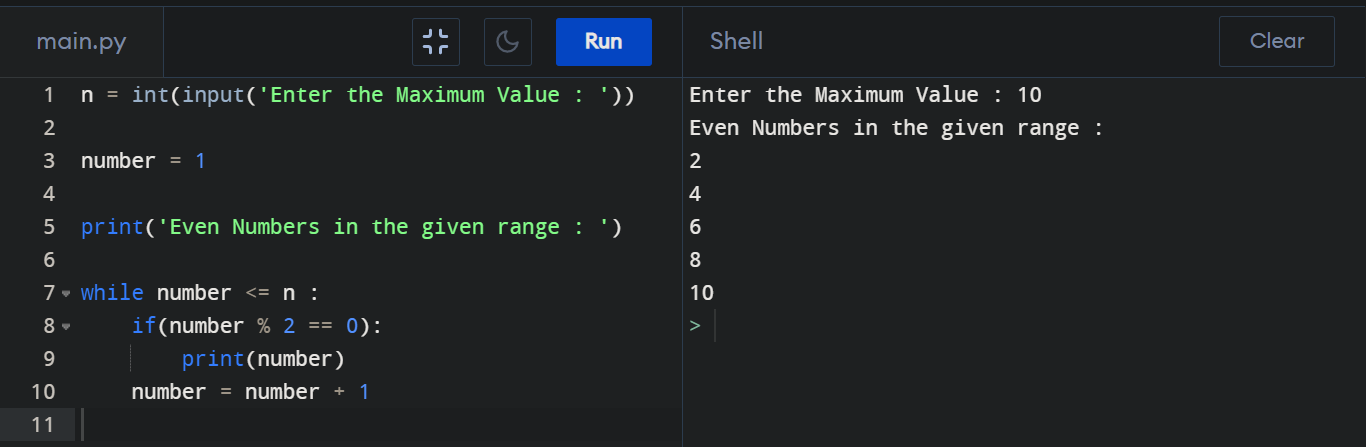Python Program to Print Even Numbers

How to print Even Numbers in a given range? We can do it by using a Loop and a Conditional Statement. Here we used "while loop" and "if statement" to get our desired output.

Printing Odd Numbers by Python :

n = int(input('Enter the Maximum Value : '))

number = 1

print('Even Numbers in the given range : ')

while number <= n :
if(number % 2 == 0):
print(number)
number = number + 1

Output :